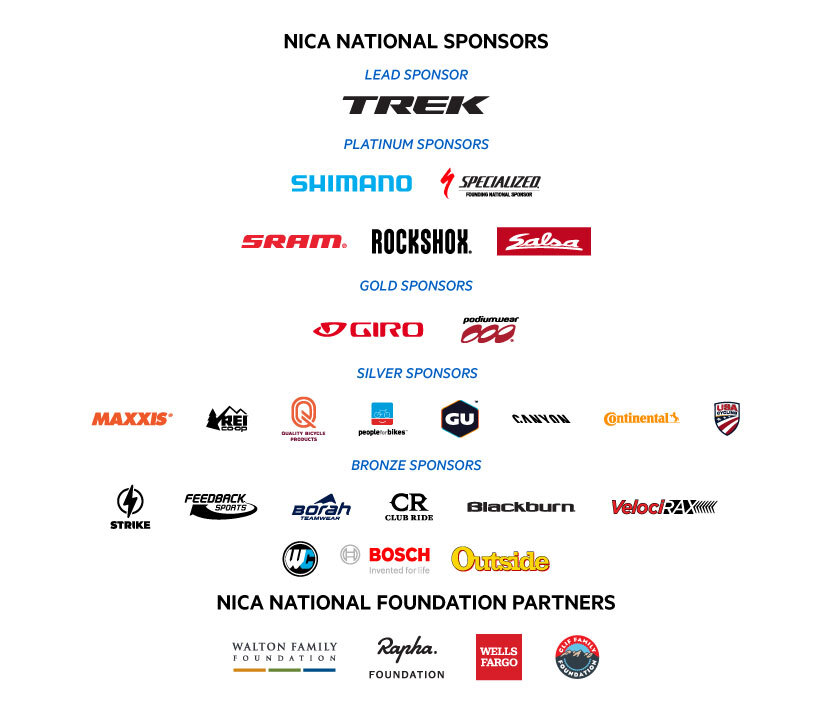Like   Tweet   Pin   +1   in
 /* styles */ Hello ! Phil Hynes and I just returned from a weekend of training new coach supporters in NorCal and I always return from NICA trips with so much admiration for NICA coaches. You all are so dedicated to your programs. Thanks for keeping NICA fun while honoring the goals of all of your student-athletes. You lead by example everyday on and off the bike. We share some great tips at the bottom of the newsletter on how to model good sportsmanship for your student-athletes. Your athletes are always watching you even when it doesn't seem like they are paying attention. Student-athletes are always the first ones to notice your new helmet or a new pair of shoes. They are also the first ones to notice your positive attitude and the way that you include all athletes without regard for their skill level. You have the ability to help young athletes grow into amazing adults. Thanks for taking the time to be a coach! -Mike McGarry and the NICA Coach Licensing Team In This Issue: - Game of the Month: Have You Ever? - NICA Course Expiration Dates and Expiration Reminder Emails - Race Day Coaching: Preparation and Reflection - Adventure Spotlight - Trek NICA Pathfinders Scholarship - Application Now Open! - NICA Celebrates Four Years of Injury Reduction! - 2022 NICA Online Leaders’ Summit Schedule - NICA Handbook Spotlight: Racing Safety Standards - Partner and Sponsor Content - GRiT Corner - 2022 Sparkle On Scholarship - TrueSport: 9 Ways to Model Good Sportsmanship from the Sidelines
 table div table+table+table div table{width:100%;padding:0}table div table+table+table div table img{width:96.23%;padding:0;float:none}table div table+table+table div table td{width:100%;padding:0 1.88% 18px}/* styles */## Game of the Month - Have You Ever?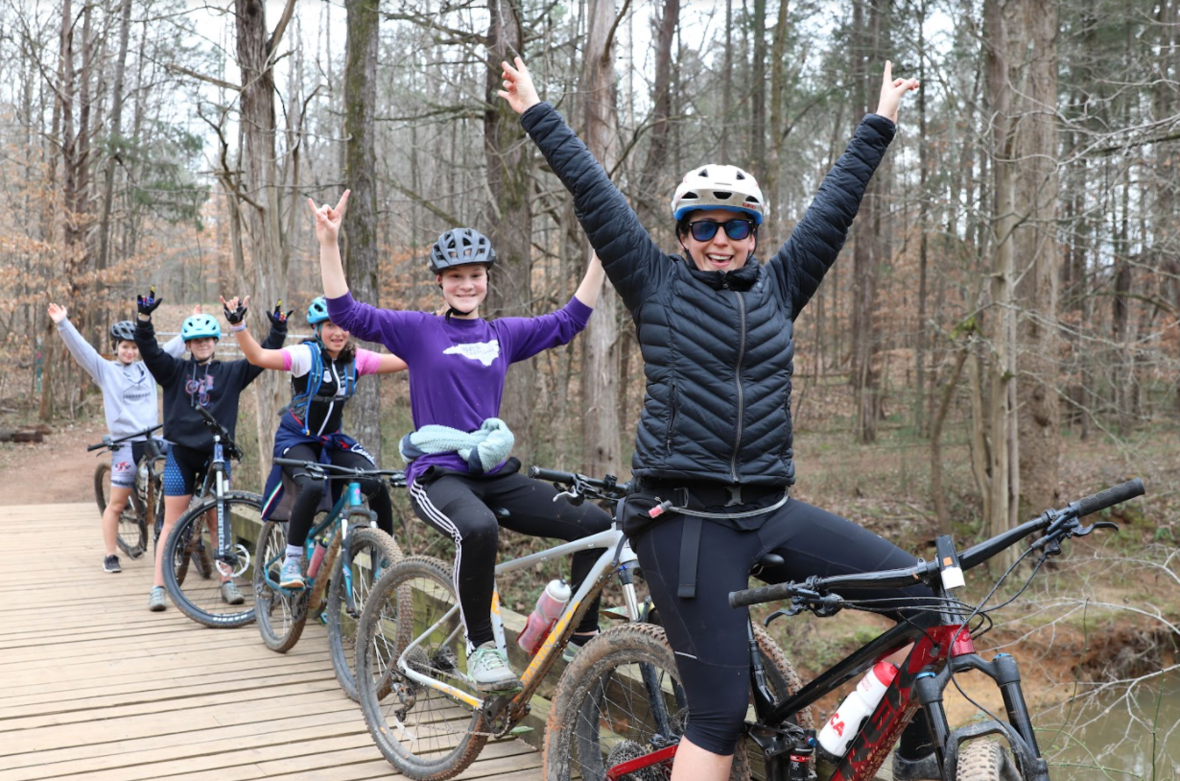Send us images of your teams playing games! Please send to coachlicensing@nationalmtb.org

Where: Open Space
Objectives: I can build a strong team culture. I can learn more about my teammates.
Setup: Have your team form a circle. You can also use cones to create a starting line. This can be done as an on the bike game by placing cones a distance away for student-athletes to ride around.
Rules:Ask one of the following questions. If your student-athlete answers yes to the question they step into the center of the circle. If it isn’t true for them, they just stand still. If forming a line, have all student-athletes stand on one side of the line. Ask them one of the following questions. If the question is true for them, they are to cross over the line. If it isn’t true for them, they just stand still. They just keep going back and forth over the line if the questions are true for them. Or if doing this as an on the bike game have them ride around the cone if they answer yes to a question.

Have You Ever...
...broken a bone mountain biking?
...been on a roller coaster?
...dug a hole in the sand until you hit water?
...actively looked for your car in a parking lot for over 15 minutes?
...called your teacher mom?
...stayed up all night?
...picked up food off the ground and ate it?
...driven a go-cart?
...given yourself a haircut?
...spent the night in a hammock?
...flown in your dreams?
...accidentally sent an inappropriate text message to your mom that was intended for a friend?
...lost your glasses and found them on your face?
...been out of the country?
...faked an illness to stay home from school?
...spent the night in the hospital?
...used someone else’s toothbrush?
...changed a baby’s diaper?
...farted at the grocery store and quickly left the aisle?
...participated in a school musical?
...seen a cassette tape?
...faked having to use the bathroom to get out of a boring class?

Reflection Question: What surprised you the most?

 table div table+table+table+table+table+table div table{width:100%;padding:0}table div table+table+table+table+table+table div table img{width:96.23%;padding:0;float:none}table div table+table+table+table+table+table div table td{width:100%;padding:0 1.88% 18px}/* styles */## Pit Zone Course Expiration Dates and Reminder Emails

 /* styles */ If you have been in the Pit Zone recently you will notice that there is an expiration date for each course that is required annually or every two years. Please don’t attempt to re-certify expiring NICA courses until: 1) You receive a reminder email from NICA Education or 2) The course status in Pit Zone is highlighted in Yellow or Red If there is a green check mark on the course status, you are good. If the status is highlighted in yellow or red, you are ready to take the course again. Please see the example attached. We understand that remembering when courses are about to expire or going into the Pit Zone to check is not always an efficient use of your time! No need to remember or check anymore. Our new NICA Coach Education platform will now automatically send you a reminder email 30 days in advance of a course expiring so you are prepared to complete the course again before it expires. Additionally, once a course has expired, NICA Education will send you a detailed email of what course has expired so you can jump right in and complete it to maintain your license. Once notified, simply log into your NICA Pit Zone account and click on the “Access Coach Courses” button to navigate to the NICA Education Center to retake the course. If you have any questions please contact us at: coachlicensing@nationalmtb.org.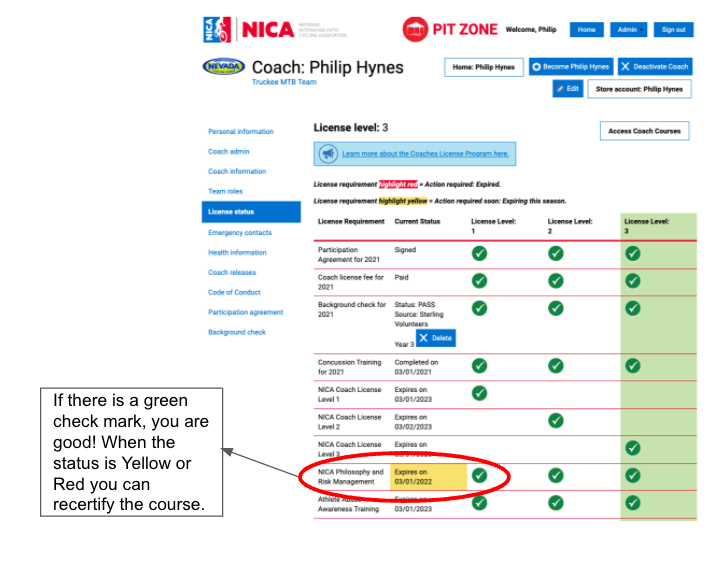If the check mark is green you are good! If the status is highlighted in Yellow or Red you need to recertify the course.
 table div table+table+table+table+table+table+table+table+table+table+table div table{width:100%;padding:0}table div table+table+table+table+table+table+table+table+table+table+table div table img{width:96.23%;padding:0;float:none}table div table+table+table+table+table+table+table+table+table+table+table div table td{width:100%;padding:0 1.88% 18px}/* styles */## Race Day Coaching: Preparation and Reflection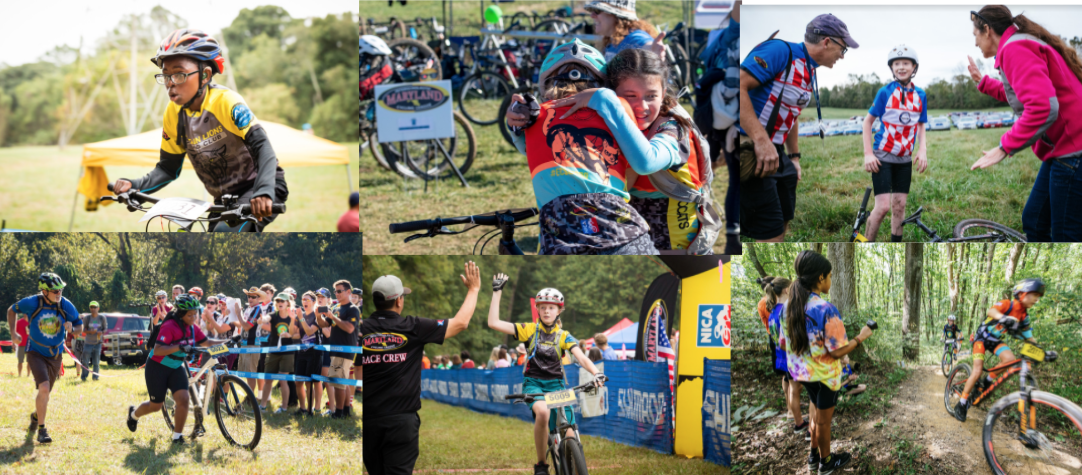NICA Coaches are integral to a student-athlete’s experience and the athlete’s enjoyment and progression in mountain biking. As coaches we have the opportunity to positively impact and the potential to negatively impact their experience. You can help your student-athletes pursue their best self through mountain biking and strengthen your relationship with them through positive and constructive communication.

As you approach Race Day, the questions below offer an opportunity for you to gain a fuller understanding of your student-athlete’s NICA experience, deepen your relationship with them, and ensure your own positive NICA team experience.

The value to these questions is in the exchange, not necessarily in the answer - there are no right or wrong answers. Keep it conversational. Ask the questions you are comfortable asking. If it doesn’t feel natural don’t force it. This is a playbook, not a script.

Questions for Before an Event/Race
Goals - What are two controllable goals you have for this race? (time & place are not controllable; eating well during the race, passing, smooth descending, pacing, staying positive are controllable)
Progress - What is something you hope to do better than you have in the past?
Learning - What have you learned in practice that will help you at this event? What piece of advice have you been given that you will use in this event?
Challenge - How will you pace yourself today? How will you handle this weather? What will be the hardest part of the course?
Performance/Success/Results - What will make this race a success for you?
Fun - What are the parts of the event that you think will make you smile the biggest?
Pride - How will you cheer on your teammates today?
Reflection - What part of the day are you thinking about the most? And why?

Questions for After an Event/Race
Goals and Progress - What were your goals today, and how did it go? Did you meet them?
Learning - What did you learn from the race? How will you use what you learned in the future?
Challenge - What was the most challenging part of the event?
Performance/Success/Results - What do you feel was your biggest success at the event?
Fun - What was the most fun at the event? Who was part of the funniest moment of the day?
Pride - Who were you most proud of at the event?
Reflection - What would you do differently?

 table div table+table+table+table+table+table+table+table+table+table+table+table+table+table div table{width:100%;padding:0}table div table+table+table+table+table+table+table+table+table+table+table+table+table+table div table img{width:96.23%;padding:0;float:none}table div table+table+table+table+table+table+table+table+table+table+table+table+table+table div table td{width:100%;padding:0 1.88% 18px}/* styles */table div table+table+table+table+table+table+table+table+table+table+table+table+table+table+table+table div table,table.module-15{width:32.08%;float:right;padding:0}table div table+table+table+table+table+table+table+table+table+table+table+table+table+table+table+table div table a{border:0 none;text-decoration:none}table div table+table+table+table+table+table+table+table+table+table+table+table+table+table+table+table div table img{width:100%!important;border:0 none;text-decoration:none}table div table+table+table+table+table+table+table+table+table+table+table+table+table+table+table+table div table td{width:100%;padding:0 0 20px 20px}/* styles */ Let’s dig into the phrase “Challenge by Choice.” The simple principle is that student-athletes are invited to voluntarily participate in activities. A student-athlete may choose to sit out of an activity and this right is to be respected by everyone in the group. Here’s an example of how this shows up at NICA practice. You roll up to a challenging rock garden that exceeds the ability of the riders in your group. No one in your group has ridden this rock garden yet and a ride around option is available. You stop your group and provide the teaching points needed to ride the more difficult line. All riders are asked to walk their bike through the most challenging line. Then you demo the skills needed and ride the rock garden. You ask your group who would like to session the section. Allow your riders to attempt whichever line they want, including the ride around.
 table div table+table+table+table+table+table+table+table+table+table+table+table+table+table+table+table+table div table,table.module-16{width:32.08%;float:left;padding:0}table div table+table+table+table+table+table+table+table+table+table+table+table+table+table+table+table+table div table a{border:0 none;text-decoration:none}table div table+table+table+table+table+table+table+table+table+table+table+table+table+table+table+table+table div table img{width:100%!important;border:0 none;text-decoration:none}table div table+table+table+table+table+table+table+table+table+table+table+table+table+table+table+table+table div table td{width:100%;padding:0 20px 20px 0}/* styles */ The key points in utilizing challenge by choice: - You provided the teaching points and demo needed for the rock garden. - You walked your riders through the rock garden. - They can have success in walking through the garden, riding the ride around option, or attempting the rock garden. Be thoughtful in giving student-athletes the choice on how they want to participate. Remember, your influence as a coach is never neutral. Be wary of your power as a coach, and continue to create opportunities for student-athlete choice.
 table div table+table+table+table+table+table+table+table+table+table+table+table+table+table+table+table+table+table div table{width:100%;padding:0}table div table+table+table+table+table+table+table+table+table+table+table+table+table+table+table+table+table+table div table img{width:96.23%;padding:0;float:none}table div table+table+table+table+table+table+table+table+table+table+table+table+table+table+table+table+table+table div table td{width:100%;padding:0 1.88% 18px}/* styles */## Trek NICA Pathfinders Scholarship - Application Now Open!

 table div table+table+table+table+table+table+table+table+table+table+table+table+table+table+table+table+table+table+table+table div table,table.module-19{width:40.94%;float:left;padding:0}table div table+table+table+table+table+table+table+table+table+table+table+table+table+table+table+table+table+table+table+table div table a{border:0 none;text-decoration:none}table div table+table+table+table+table+table+table+table+table+table+table+table+table+table+table+table+table+table+table+table div table img{width:100%!important;border:0 none;text-decoration:none}table div table+table+table+table+table+table+table+table+table+table+table+table+table+table+table+table+table+table+table+table div table td{width:100%;padding:0 20px 20px 0}/* styles */ We are excited to announce that applications are now open for Trek NICA Pathfinders Scholarships for our Fall Leagues! Trek has partnered with NICA to grow diversity in the sport of cycling and provide better access for people of color. This scholarship will provide up to 250 diverse and underrepresented youth with the gear and funds they need to ride and race in their local NICA League. If you know a student-athlete who would be a good fit, learn more and apply here! See the story of Genesis, a Park City, Utah teen and Pathfinders recipient whose life has been transformed through greater access to the outdoors, here.
 table div table+table+table+table+table+table+table+table+table+table+table+table+table+table+table+table+table+table+table+table+table div table{width:100%;padding:0}table div table+table+table+table+table+table+table+table+table+table+table+table+table+table+table+table+table+table+table+table+table div table img{width:96.23%;padding:0;float:none}table div table+table+table+table+table+table+table+table+table+table+table+table+table+table+table+table+table+table+table+table+table div table td{width:100%;padding:0 1.88% 18px}/* styles */## NICA Celebrates Four Years of Injury Reduction!

 table div table+table+table+table+table+table+table+table+table+table+table+table+table+table+table+table+table+table+table+table+table+table+table div table,table.module-22{width:32.08%;float:right;padding:0}table div table+table+table+table+table+table+table+table+table+table+table+table+table+table+table+table+table+table+table+table+table+table+table div table a{border:0 none;text-decoration:none}table div table+table+table+table+table+table+table+table+table+table+table+table+table+table+table+table+table+table+table+table+table+table+table div table img{width:100%!important;border:0 none;text-decoration:none}table div table+table+table+table+table+table+table+table+table+table+table+table+table+table+table+table+table+table+table+table+table+table+table div table td{width:100%;padding:0 0 20px 20px}/* styles */ Since 2018 when NICA and the University of Utah launched the NICA Safety Reporting and Study Partnership, we’ve seen an injury-rate reduction for Student-Athletes by 38% and Coaches by 25%. While injuries are inherent to participation in NICA as with any sports organization, we strive to minimize potential for injuries. To continue on our path of injury reduction, please keep practicing fundamental skills among other safety best practices (see link to flier below).
 table div table+table+table+table+table+table+table+table+table+table+table+table+table+table+table+table+table+table+table+table+table+table+table+table+table div table{width:100%;padding:0}table div table+table+table+table+table+table+table+table+table+table+table+table+table+table+table+table+table+table+table+table+table+table+table+table+table div table img{width:96.23%;padding:0;float:none}table div table+table+table+table+table+table+table+table+table+table+table+table+table+table+table+table+table+table+table+table+table+table+table+table+table div table td{width:100%;padding:0 1.88% 18px}/* styles */## 2022 NICA Online Leaders’ Summit Schedule

This year, the NICA Coach Licensing Staff is offering 9 full weeks of live, online Leaders’ Summit sessions so that you can get the necessary core requirements for your Level 3 Coach License or the continuing education units needed to maintain your current coach license. Please see below for details about the online leader summit program:

▪ The schedule with registration links is listed below.
▪ Online Leaders Summits will be held live via Zoom video conference.
▪ Sessions will be presented by NICA Coach Licensing staff.
▪ They will consist of the 5 Core Required sessions to get your Coach License Level 3 or count as CEUs to maintain your current Coach License Level 2 or 3.
▪ Sessions will be offered in 5 one hour sessions on weekdays. Times will vary each week to accommodate your schedule as best we can.
▪ Please use the same email address you use for Pit Zone to register for the Zoom sessions. (If you use a different email automatic updates to your Pit Zone cannot happen)
▪ Pre-registration is required to attend each online leader summit session.
 ▪ The schedule with registration links is listed below.
 ▪ Online Leaders Summits will be held live via Zoom video conference.
 ▪ Sessions will be presented by NICA Coach Licensing staff.
 ▪ They will consist of the 5 Core Required sessions to get your Coach License Level 3 or count as CEUs to maintain your current Coach License Level 2 or 3.
 ▪ Sessions will be offered in 5 one hour sessions on weekdays. Times will vary each week to accommodate your schedule as best we can.
 ▪ Please use the same email address you use for Pit Zone to register for the Zoom sessions. (If you use a different email automatic updates to your Pit Zone cannot happen)
 ▪ Pre-registration is required to attend each online leader summit session.
 table div table+table+table+table+table+table+table+table+table+table+table+table+table+table+table+table+table+table+table+table+table+table+table+table+table+table+table+table div table td,table.module-27{width:100%;padding:0}table div table+table+table+table+table+table+table+table+table+table+table+table+table+table+table+table+table+table+table+table+table+table+table+table+table+table+table+table div table{width:100%;float:none;margin-left:auto;margin-right:auto;padding:0}table div table+table+table+table+table+table+table+table+table+table+table+table+table+table+table+table+table+table+table+table+table+table+table+table+table+table+table+table div table a{border:0 none;text-decoration:none}table div table+table+table+table+table+table+table+table+table+table+table+table+table+table+table+table+table+table+table+table+table+table+table+table+table+table+table+table div table img{width:100%!important;border:0 none;text-decoration:none}/* styles */
 table div table+table+table+table+table+table+table+table+table+table+table+table+table+table+table+table+table+table+table+table+table+table+table+table+table+table+table+table+table+table div table{width:100%;padding:0}table div table+table+table+table+table+table+table+table+table+table+table+table+table+table+table+table+table+table+table+table+table+table+table+table+table+table+table+table+table+table div table img{width:96.23%;padding:0;float:none}table div table+table+table+table+table+table+table+table+table+table+table+table+table+table+table+table+table+table+table+table+table+table+table+table+table+table+table+table+table+table div table td{width:100%;padding:0 1.88% 18px}/* styles */## NICA Handbook Spotlight: Racing Safety Standards

 /* styles */ SECTION 6.10: RACING SAFETY STANDARDS 6.10.A PRE-RIDE PROVIDED WHEN POSSIBLE Student-athletes will be given the opportunity to walk the course and participate in a practice ride whenever possible. 6.10.B Pre-Ride is a regularly scheduled practice ride on the race course that takes place on the same weekend as the race. For practice rides on race courses at other times, the Pre-Ride Rules do not apply.
 table div table+table+table+table+table+table+table+table+table+table+table+table+table+table+table+table+table+table+table+table+table+table+table+table+table+table+table+table+table+table+table+table+table div table,table.module-32{width:39.43%;float:right;padding:0}table div table+table+table+table+table+table+table+table+table+table+table+table+table+table+table+table+table+table+table+table+table+table+table+table+table+table+table+table+table+table+table+table+table div table a{border:0 none;text-decoration:none}table div table+table+table+table+table+table+table+table+table+table+table+table+table+table+table+table+table+table+table+table+table+table+table+table+table+table+table+table+table+table+table+table+table div table img{width:100%!important;border:0 none;text-decoration:none}table div table+table+table+table+table+table+table+table+table+table+table+table+table+table+table+table+table+table+table+table+table+table+table+table+table+table+table+table+table+table+table+table+table div table td{width:100%;padding:0 0 20px 20px}/* styles */ 6.10.C. RACERS AND COACHES ONLY ON THE COURSE 6.10.C.1 Only student-athletes entered for competition in the event and their team coaches may ride the designated race course at times when no race is currently taking place. An event is taking place at all times after the start of a race until the time the last rider in that race finishes. No coach is permitted to ride the course during an event. 6.10.C.2 A violation of this rule will result in a team-level penalty against the associated team of the offender: a. First Offense: 25-point penalty deducted from team score on race day. b. Second offense: 50-point penalty deducted from team score on race day. c. Third offense: 100-point penalty deducted from team score on race day. 6.10.D. NUMBER PLATES ARE MANDATORY Once number plates are distributed, students must have them properly affixed to their bicycles at all times they are on the course.
 table div table+table+table+table+table+table+table+table+table+table+table+table+table+table+table+table+table+table+table+table+table+table+table+table+table+table+table+table+table+table+table+table+table+table div table{width:100%;padding:0}table div table+table+table+table+table+table+table+table+table+table+table+table+table+table+table+table+table+table+table+table+table+table+table+table+table+table+table+table+table+table+table+table+table+table div table img{width:96.23%;padding:0;float:none}table div table+table+table+table+table+table+table+table+table+table+table+table+table+table+table+table+table+table+table+table+table+table+table+table+table+table+table+table+table+table+table+table+table+table div table td{width:100%;padding:0 1.88% 18px}/* styles */## Partner and Sponsor Content

 table div table+table+table+table+table+table+table+table+table+table+table+table+table+table+table+table+table+table+table+table+table+table+table+table+table+table+table+table+table+table+table+table+table+table+table+table div table,table.module-35{width:22.26%;float:left;padding:0}table div table+table+table+table+table+table+table+table+table+table+table+table+table+table+table+table+table+table+table+table+table+table+table+table+table+table+table+table+table+table+table+table+table+table+table+table div table a{border:0 none;text-decoration:none}table div table+table+table+table+table+table+table+table+table+table+table+table+table+table+table+table+table+table+table+table+table+table+table+table+table+table+table+table+table+table+table+table+table+table+table+table div table img{width:100%!important;border:0 none;text-decoration:none}table div table+table+table+table+table+table+table+table+table+table+table+table+table+table+table+table+table+table+table+table+table+table+table+table+table+table+table+table+table+table+table+table+table+table+table+table div table td{width:100%;padding:0 20px 20px 0}/* styles */ Strike now!!! Strike Visulas has some great PROMOS going on. Check them out and get ready for your races!
 table div table+table+table+table+table+table+table+table+table+table+table+table+table+table+table+table+table+table+table+table+table+table+table+table+table+table+table+table+table+table+table+table+table+table+table+table+table div table{width:100%;padding:0}table div table+table+table+table+table+table+table+table+table+table+table+table+table+table+table+table+table+table+table+table+table+table+table+table+table+table+table+table+table+table+table+table+table+table+table+table+table div table img{width:96.23%;padding:0;float:none}table div table+table+table+table+table+table+table+table+table+table+table+table+table+table+table+table+table+table+table+table+table+table+table+table+table+table+table+table+table+table+table+table+table+table+table+table+table div table td{width:100%;padding:0 1.88% 18px}/* styles */table div table+table+table+table+table+table+table+table+table+table+table+table+table+table+table+table+table+table+table+table+table+table+table+table+table+table+table+table+table+table+table+table+table+table+table+table+table+table div table td,table.module-37{width:100%;padding:0}table div table+table+table+table+table+table+table+table+table+table+table+table+table+table+table+table+table+table+table+table+table+table+table+table+table+table+table+table+table+table+table+table+table+table+table+table+table+table div table{width:100%;float:none;margin-left:auto;margin-right:auto;padding:0}table div table+table+table+table+table+table+table+table+table+table+table+table+table+table+table+table+table+table+table+table+table+table+table+table+table+table+table+table+table+table+table+table+table+table+table+table+table+table div table a{border:0 none;text-decoration:none}table div table+table+table+table+table+table+table+table+table+table+table+table+table+table+table+table+table+table+table+table+table+table+table+table+table+table+table+table+table+table+table+table+table+table+table+table+table+table div table img{width:100%!important;border:0 none;text-decoration:none}/* styles */
 table div table+table+table+table+table+table+table+table+table+table+table+table+table+table+table+table+table+table+table+table+table+table+table+table+table+table+table+table+table+table+table+table+table+table+table+table+table+table+table div table,table.module-38{width:39.06%;float:right;padding:0}table div table+table+table+table+table+table+table+table+table+table+table+table+table+table+table+table+table+table+table+table+table+table+table+table+table+table+table+table+table+table+table+table+table+table+table+table+table+table+table div table a{border:0 none;text-decoration:none}table div table+table+table+table+table+table+table+table+table+table+table+table+table+table+table+table+table+table+table+table+table+table+table+table+table+table+table+table+table+table+table+table+table+table+table+table+table+table+table div table img{width:100%!important;border:0 none;text-decoration:none}table div table+table+table+table+table+table+table+table+table+table+table+table+table+table+table+table+table+table+table+table+table+table+table+table+table+table+table+table+table+table+table+table+table+table+table+table+table+table+table div table td{width:100%;padding:0 0 20px 20px}/* styles */ We look closely at our numbers to try to understand trends and what works best in terms of getting (and keeping) more girls on bikes. One clear correlation is that having more female coaches helps us recruit and retain more female student-athletes. Why? It’s not necessarily about the coaching–we have many male coaches who are amazing at working with both male and female student athletes. However, for new riders thinking of joining a team or the sport, it’s important for them to have role models and to see themselves represented. As Marian Wright Edelman famously said, “You can’t be what you can’t see.” So for girls and people of color, who are tremendously underrepresented in mountain biking, it is critical to have women and people of color as coaches. Their presence as coaches creates a sense of permission and invitation for girls and other underrepresented groups to join the sport. Their presence grants “permission” simply by modeling participation and showing that women and people of color belong in mountain biking. And their presence as coaches invites participation by promising a safe space for new riders to try it out. That is why GRiT is about far more than getting #moregirlsonbikes. In order to really build, strengthen and diversify our leagues, we must get more women and people of color on bikes as coaches. Those coaches can then help us reach and recruit more girls and student-athletes of color. Stay tuned over the next couple of months for more on how to recruit and support your female coach community and how male coaches can serve as allies, helping to get more girls and women on bikes! In the meantime, please reach out to Emily at egreen@nationalmtb.org if you’d like to strategize about how to do this on your team.
 table div table+table+table+table+table+table+table+table+table+table+table+table+table+table+table+table+table+table+table+table+table+table+table+table+table+table+table+table+table+table+table+table+table+table+table+table+table+table+table+table div table{width:100%;padding:0}table div table+table+table+table+table+table+table+table+table+table+table+table+table+table+table+table+table+table+table+table+table+table+table+table+table+table+table+table+table+table+table+table+table+table+table+table+table+table+table+table div table img{width:96.23%;padding:0;float:none}table div table+table+table+table+table+table+table+table+table+table+table+table+table+table+table+table+table+table+table+table+table+table+table+table+table+table+table+table+table+table+table+table+table+table+table+table+table+table+table+table div table td{width:100%;padding:0 1.88% 18px}/* styles */## 2022 Sparkle on Scholarships - Coming SoonCalling all Seniors to Save the Date! The Sparkle On Scholarship presented by Kate Courtney in partnership with SCOTT, Syncros, SRAM, and RockShox recognizes and supports student-athletes who have demonstrated a commitment to education and the cycling community during their time with NICA and will soon attend college. This year, Sparkle On will be awarded to six NICA seniors who show determination in pursuit of academic and athletic goals and a commitment to the cycling community and intend to continue to support and participate in cycling in college. The scholarships will include a monetary award of \$6,500 each, mentorship in the form of quarterly calls with Kate, and participation in a Summit focused on skill building, on and off the bike. The application period will open on March 1 and close on March 21, 2022. Details to be shared via the NICA National website soon!

 table div table+table+table+table+table+table+table+table+table+table+table+table+table+table+table+table+table+table+table+table+table+table+table+table+table+table+table+table+table+table+table+table+table+table+table+table+table+table+table+table+table+table+table div table{width:100%;padding:0}table div table+table+table+table+table+table+table+table+table+table+table+table+table+table+table+table+table+table+table+table+table+table+table+table+table+table+table+table+table+table+table+table+table+table+table+table+table+table+table+table+table+table+table div table img{width:96.23%;padding:0;float:none}table div table+table+table+table+table+table+table+table+table+table+table+table+table+table+table+table+table+table+table+table+table+table+table+table+table+table+table+table+table+table+table+table+table+table+table+table+table+table+table+table+table+table+table div table td{width:100%;padding:0 1.88% 18px}/* styles */## TrueSport: 9 Ways to Model Good Sportsmanship from the Sidelines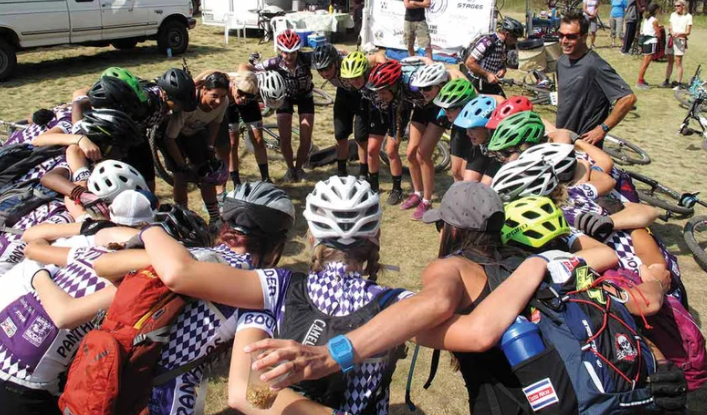table div table+table+table+table+table+table+table+table+table+table+table+table+table+table+table+table+table+table+table+table+table+table+table+table+table+table+table+table+table+table+table+table+table+table+table+table+table+table+table+table+table+table+table+table+table+table+table+table div table{width:100%;padding:0}table div table+table+table+table+table+table+table+table+table+table+table+table+table+table+table+table+table+table+table+table+table+table+table+table+table+table+table+table+table+table+table+table+table+table+table+table+table+table+table+table+table+table+table+table+table+table+table+table div table img{width:96.23%;padding:0;float:none}table div table+table+table+table+table+table+table+table+table+table+table+table+table+table+table+table+table+table+table+table+table+table+table+table+table+table+table+table+table+table+table+table+table+table+table+table+table+table+table+table+table+table+table+table+table+table+table+table div table td{width:100%;padding:0 1.88% 18px}/* styles */